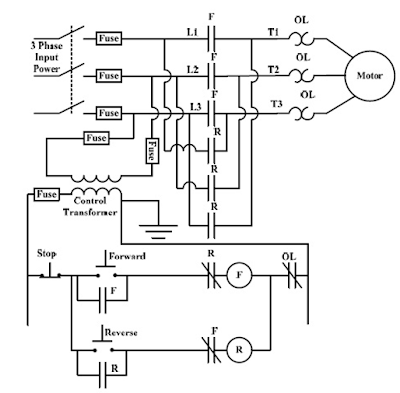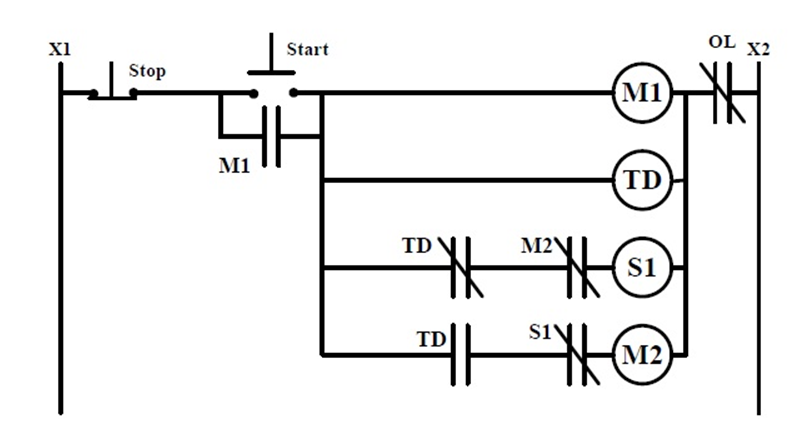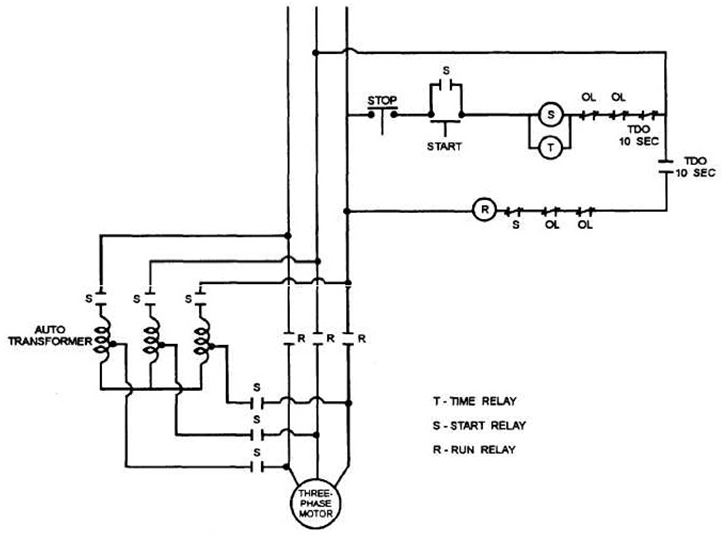### Introduction

Motor controller is a device or group of devices that serves to govern in some predetermined manner the performance of an electric motor.

A motor controller includes a manual or automatic means for starting or stopping a motor, selecting a forward or reverse rotation, selecting and regulating a speed, regulating or limiting the torque and protecting against overloads and faults.

In short industrial motor control are essential to the operation of an electric motor.

### Control Circuit and Power Circuit

In electric motor control we are dealing with two types of circuits namely the control circuit and the power circuit.
• Control Circuit - the circuit that governs the action of control  elements such as magnetic contactor, push button, overload relay, limit switch, auxiliary contacts of protection devices and others.
• Power Circuit - this is the circuit that directly control the flow of electrical energy from the source to the load.
Control circuit and power circuit are totally independent with each other and they can be both installed in one application with different voltages. Some industries use 440 VAC as the supply voltage for their electric motors while using 24 VDC to supply their control circuits.Figure 1. Control Circuit and Power Circuit

The above diagram describes how control circuit and power circuit interact with each other.
• In that figure, when the switch S is close it will energize the relay coil which is connected in series with that switch.
• In effect the auxiliary contact M1 will also close due to the magnetic action of the coil of the relay.
• And obviously the motor which is connected in parallel to the 480 VAC supply will also be energize.
The given example used a method where the control circuit and the power circuit have the same voltage supply which is 480 Volts AC.

Now imagine that the path taken by the switch and the relay coil is supplied by another source, is it possible? The answer is yes all we have to do is to change the specification of the relay coil to be used from 480 VAC to the new supply voltage i.e. 24 VDC or 110 VAC.Figure 2. Power Circuit and Control Circuit have different supply voltage

The circuit in figure 2 describe a certain case where the control circuit and power circuit have different supply voltage. Motor control designers have different views in choosing the method they want. For convenience and practicality some engineers opted that the two circuits have similar supply while others on the contrary since they avoid the risk of humming effect as a result of supplying AC voltage to the contactor. The latter used DC supply and of course DC voltage contactor.

#### 1. Full Voltage Starting

The most common type of motor starter is full voltage starter, some people call this method as a direct-across-the-line or direct-on-line (DOL) motor starter.Figure 3. Full Voltage Motor Starter

This is used to control motors in one direction and in using this the starting current will reach as high as 600% of it's full load current.

Example:

A 3 phase 20 HP motor that is connected to a supply voltage of 220 VAC @ 80% pf. Find the starting current when it is connected to a full voltage starter. (Use 80% pf and 85% efficiency)

Solution:
• IFL = (20 HP x 746) / [ sqrt (3) x 220 x 0.8 x 0.85) ] = 58 Amperes
• Starting current = IFL  *  600% = 345 Amperes
• This starting current will last for  3-5 seconds
This method of starting is cheap and easy to install but it has a detrimental effect when used in motors larger than 10 HP. This type of starter is commonly used in small conveyors, pump and air-conditioning unit.

#### 2. Full voltage starter reversing

Commonly known as forward-reverse, this motor starter similarly used full voltage starting method but it can control the motor in two directions.Figure 3. Forward-Reverse (photo: www.pdhengineer.com)

• In this case there are two magnetic contactors, the forward contactor F and the reverse contactor R.
• The key for reversing the direction of the motor is  to interchange lines L1 and L3 during the time that the R contactor activates.
• The two normally closed contacts that are connected in series in F and R rung respectively are called electrical interlocks. This is to prevent the simultaneous activation of the 2 rungs that might cause serious damage to the equipments if happen.
Similar to non-reversing full voltage starter, this is practical when use for motors lower than 10 HP. Since it is reversing this is commonly used in applications that needs reversing action such as dumb waiter, overhead cranes, reversing conveyor and others.

#### How to determine the contactor sizes?

We can determine the contactor sizes for full voltage starter by first determining the motor continuous current. Continuous ampere rating should always more than the rated current or the full load current.

We can derive the maximum continuous current based on the design of the motor. Some NEMA AC motors have service factor of 1.35. That means that motor is allowed (by design) to run more than its full load capacity without being damaged. For example a 10 HP with a service factor of 1.35 can supply a load up to 13.5 HP without being damage. Therefore we can base the contactor rating in consonance to the service factor to expect that such a motor would serve that overload.

Example:

A 3 phase 20 HP motor that is connected to a supply voltage of 220 VAC @ 80% pf. Find the maximum continuous ampere rating if that motor has a service factor of 1.35. (Use 80% pf and 85% efficiency)

Solution:
• IFL = (20 HP x 746) / [ sqrt (3) x 220 x 0.8 x 0.85) ] = 58 Amperes
• Apply service factor = 1.35 x 58 amperes = 78.3 Amperes
Therefore we can set the maximum continuous current to 78.3 Ampere. And after getting the maximum ampere rating, check the table below for right NEMA size of the contactor to be used to served that load.

 NEMA CONTACTOR SIZES Maximum HP Rating for Each Voltage Category NEMA SIZE Continuous Amp. Rating Single Phase Three phase 115 V 230 V 208 V 240 V 480 V 600 V 00 9 1/3 1 1-1/2 1-1/2 2 2 0 18 1 2 3 3 5 5 1 27 2 7-1/2 7-1/2 7-1/2 10 10 2 45 10 15 25 25 3 90 25 30 50 50 4 135 40 50 100 100 5 270 75 100 200 200 6 540 150 200 400 400 7 810 200 300 600 600 8 1215 400 450 900 900
Table 1. NEMA Contactor Sizes

#### 3. Reduced Voltage: Wye-Delta

Wye-Delta or star-delta motor starting methods is a type of reduce voltage starting method where the 3 phase motor is connected first to WYE or STAR then after 3-5 seconds it will be connected to DELTA. This can be accomplish through actions in the magnetic contactor.

During starting period the line voltage  that supplies the motor is still the same but the voltage across the each phase of the motor coil is reduced. Thus,

• Starting Voltage Reduction = Line Voltage / sqrt (3)
• For example the motor rated 440 V has a starting voltage cross each coil of the three phase motor of 440 V/ 1.73 = 254 Volts.Figure 4. Wye-Delta Control Circuit (photo: www.pdhengineer.com)
• When the start button is press the main coil M1, timer TD and starting coil S1 (WYE) are activated.
• The auxiliary contact M1 is latched therefore the circuit is energized until the stop button is press.
• Right from the time the timer TD is activated it will count automatically until it reaches its pre- determined value i.e. 3-5 seconds.
• The auxiliary contact of the timer TD will change its initial state once the pre- determined time has been reached.
• For example the predetermined time is 3 seconds then during that time the normally closed contact of TD will open and at the same time the normally open contact of TD will closed. This is the time where the starting coil S1 (WYE) will be deactivated while the running coil M2 (DELTA) will be activated.
• The whole circuit will shutdown once the stop button is pressed or the overload contact in the right most side will open due to overload condition.

 Starting Methods Comparison Method % Voltage Start during starting % Full load starting torque % Full load Rated Current Full Voltage 100 100 600 Wye-Delta 58 33 200
Table 2. Comparison of starting methods

In effect the load rated ampere will also reduce since the voltage applied across the coil is also reduced, thus
• Starting Current = Full Load Ampere x 200%
• If we have motor that has  FLA of 52 Ampere, then SRC = 52 x 200% = 104 Amperes
• 104 Amperes is very much lower compare to 308 Ampere when full voltage starting is use.
The reduction of the torque would be approximately equal to the square of the reduction of the voltage across motor winding, thus
• Starting toque reduction = %V ^2 = .58 ^2 = 33% of full load torque

#### 4. Reduced Voltage: Auto-transformerFigure 5. Auto-transformer schematic diagram
Auto-transformer is kind of transformer with only one winding. Auto-transformer reduce the voltage level of one circuit to the other thru taps. Different taps have different voltage value measured from the common end of both primary and secondary.Figure 5. Auto transformer method of starting
Auto-transformer motor starter used auto-transformer to suppressed the effect of high starting current. The auto-transformer have commonly three tap settings: 50%, 65% and 80% of the full voltage. The result of the following settings are as follows:

#### % Voltage % Torque % Rated Current 50 45 300 65 76 390 80 115 480 Table 3. Effect of auto transformer starting (Rockwell Automation)

The control sequence of auto-transformer is almost similar to wye-delta but the auto-transformer is more flexible compare to wye-delta. Wye-delta is tagged at 58% reduction of starting current while auto-transformer is flexible based on the requirement.

• Auto-transformer needs only 3 leads to operate and it is advisable for installations in submersible pump where the motor is at least 100 ft. from the MCC. WYE-DELTA for submersible pump operation  is disadvantageous since it needs at least 6 leads to operate. Therefore, we can reduce 50% for the cost of the conductors in choosing auto-transformer over wye-delta.
• Auto-transformer is flexible since it can be set at 50%, 65% or 80% while wye-delta on the other hand is tagged at 58%.
1.2.